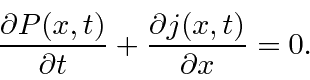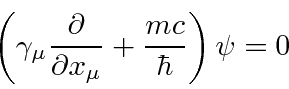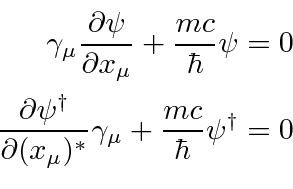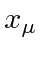## The Conserved Probability Current

We now return to the nagging problem of the probability density and current which prompted Dirac to find an equation that is first order in the time derivative. We derived the equation showing conservation of probability for 1D Schrödinger theory by using the Schrödinger equation and its complex conjugate to get an equation of the formWe also extended it to three dimensions in the same way.

Our problem to find a similar probability and flux for Dirac theory is similar but a little more difficult. Start with the Dirac equation.Since the wave function is a 4 component spinor, we will use the Hermitian conjugate of the Dirac equation instead of the complex conjugate. Thematrices are Hermitian.The complex conjugate does nothing to the spatial component ofbut does change the sign of the fourth component. To turn this back into a 4-vector expression, we can change the sign back by multiplying the equation by(from the right).Defining, the adjoint spinor, we can rewrite the Hermitian conjugate equation.This is the adjoint equation. We now multiply the Dirac equation byfrom the left and multiply the adjoint equation byfrom the right, and subtract.We have found a conserved current. Some interpretation will be required as we learn more about the solutions to the Dirac equation and ultimately quantize it. We may choose an overall constant to set the normalization. The fourth component of the current should betimes the probability density so that the derivative with respect toturns into. Therefore let us set the properly normalized conserved 4-vector to beJim Branson 2013-04-22